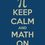# Intuitive Maths

There have been many cases of scientists finding solutions to problems in their dreams. Some have stumbled upon the required solution by their intuitive approach. I firmly believe that this method leads to discovering new things... things which are hard to explain by pure logic. One such problem is:

There is a 100m long stick lying on positive x-axis with one end on $x=0$ and there are 101 ants placed on this stick such that there is one ant on $x=0$, $x=1$, $x=2$...$x=100$. The first ant is facing right, the second is facing left, the third right, the fourth left and so on. All these ants now start walking in the direction they are facing with speed $1 m/s$. Whenever 2 ants collide, they exchange their directions immediately (but speed remain the same) and continue moving.Ants fall-off the stick once the move beyond end of the stick. Find the time taken for all the ants to leave the stick.

I am not going to reveal the answer. At first I thought, "this is a very tough question". But then the solution was extremely elegant and the motivation for it was intuition... and not hardcore maths. (Although the actual solution does involve math).

I recently stumbled upon this video and it rekindled in me, the idea of intuition. I will state a mathematical proof here. Can someone explain this in a non mathematical form?

Consider $S_1=1-1+1-1+1....$

now $S_1= 1-1+1-1+1...$ (i.e shift the 1's to the right)

adding both gives $2S_1=1\Rightarrow S_1=\frac{1}{2}$

Now consider $S_2=1-2+3-4+5...$

now, $S_2= 1-2+3-4+5...$ (i.e. shift the numbers to the right)

adding both gives $2S_2=1-1+1-1...=S_1=\frac{1}{2}\Rightarrow S_2=\frac{1}{4}$

Now consider $S_3=1+2+3+4...$

now, $S_2=1-2+3-4...$

We have $S_3-S_2=0+4+0+8+0+12...$

or $S_3-\frac{1}{4}=4+8+12....=4\times(1+2+3...)=4S_3$

$\Rightarrow -\frac{1}{4}=3S_3\Rightarrow S_3=-\frac{1}{12}$Note by Keshav Kumar
7 years, 2 months ago

This discussion board is a place to discuss our Daily Challenges and the math and science related to those challenges. Explanations are more than just a solution — they should explain the steps and thinking strategies that you used to obtain the solution. Comments should further the discussion of math and science.

When posting on Brilliant:

• Use the emojis to react to an explanation, whether you're congratulating a job well done , or just really confused .
• Ask specific questions about the challenge or the steps in somebody's explanation. Well-posed questions can add a lot to the discussion, but posting "I don't understand!" doesn't help anyone.
• Try to contribute something new to the discussion, whether it is an extension, generalization or other idea related to the challenge.
• Stay on topic — we're all here to learn more about math and science, not to hear about your favorite get-rich-quick scheme or current world events.

MarkdownAppears as
*italics* or _italics_ italics
**bold** or __bold__ bold
- bulleted- list
• bulleted
• list
1. numbered2. list
1. numbered
2. list
Note: you must add a full line of space before and after lists for them to show up correctly
paragraph 1paragraph 2

paragraph 1

paragraph 2

[example link](https://brilliant.org)example link
> This is a quote
This is a quote
    # I indented these lines
# 4 spaces, and now they show
# up as a code block.

print "hello world"
# I indented these lines
# 4 spaces, and now they show
# up as a code block.

print "hello world"
MathAppears as
Remember to wrap math in $$ ... $$ or $ ... $ to ensure proper formatting.
2 \times 3 $2 \times 3$
2^{34} $2^{34}$
a_{i-1} $a_{i-1}$
\frac{2}{3} $\frac{2}{3}$
\sqrt{2} $\sqrt{2}$
\sum_{i=1}^3 $\sum_{i=1}^3$
\sin \theta $\sin \theta$
\boxed{123} $\boxed{123}$

## Comments

Sort by:

Top Newest

What do you want to be proved?

- 5 years, 2 months ago

Log in to reply

×

Problem Loading...

Note Loading...

Set Loading...